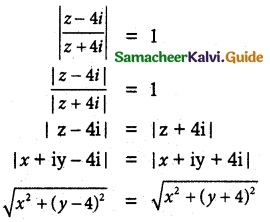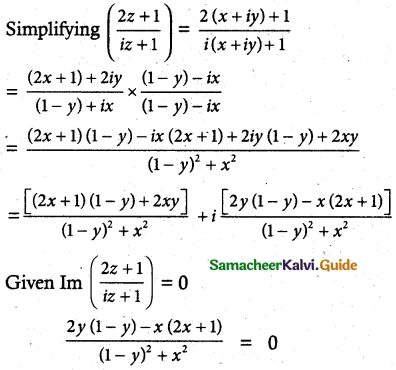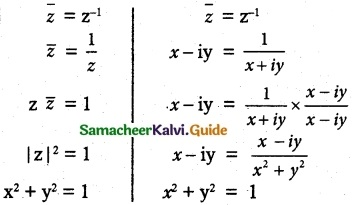Tamilnadu State Board New Syllabus Samacheer Kalvi 12th Maths Guide Pdf Chapter 2 Complex Numbers Ex 2.6 Textbook Questions and Answers, Notes.

## Tamilnadu Samacheer Kalvi 12th Maths Solutions Chapter 2 Complex Numbers Ex 2.6

Question 1.
If 2 = x + iy is a complex number such that $$\left|\frac{z-4 i}{z+4 i}\right|$$ = 1 show that the locus of z is real axis.
Solution:
Let z = x + iySquaring on both sides
x² + (y – 4)² =  x² + (y + 4)²
simplifying
We get y = 0
Which is real axis

Question 2.
If z = x + iy is a complex number such that Im $$\left(\frac{2 z+1}{i z+1}\right)$$ = 0, show that the locus of z is 2x² + 2y² + x – 2y = 0.
Solution:
Let z = x + iy2y(1 – y) – x(2x + 1) = 0
⇒ 2y – 2y² – 2x² – x = 0
∴ The locus is 2x² + 2y² – 2y + x = 0
Hence provedQuestion 3.
Obtain the Cartesian form of the locus of z = x + iy in each of the following cases:
(i) [Re (iz)]² = 3
Solution:
z = x + iy
[Re(iz)]2 = 3
⇒ [Re[i(x + iy]]2 = 3
⇒ [Re(ix – y)]2 = 3
⇒ (-y)2 = 3
⇒ y2 = 3

(ii) Im[(1 – i)z + 1] = 0
⇒ Im [(1 – i)(z + iy) + 1] = 0
⇒ Im[x + iy – ix + y + 1] = 0
⇒ Im[(x + y + 1) + i(y – x)] = 0
Considering only the imaginary part
y – x = 0 ⇒ x = y(iii) |z + i| = |z – 1|
⇒ |x + iy + i| = | x + iy – 1|
⇒ |x + i(y + 1)| = |(x – 1) + iy|
Squaring on both sides
|x + i(y + 1)|2 = |(x – 1) + iy|2
⇒ x2 + (y + 1)2 = (x – 1)2 + y2
⇒ x2 + y2 + 2y + 1 = x2 – 2x + 1 + y2
⇒ 2y + 2x = 0
⇒ x + y = 0

(iv) $$\bar {z}$$ = z-1
Solution:x² + y² = 1, x² + y² = -1 which cannot be true.
∴ x² + y² = 1Question 4.
Show that the following equations represent a circle, and, find its centre and radius.
(i) |z – 2 – i| = 3
Solution:
Let z = x + iy
|z – 2 – i| = 3
⇒ |x + iy – 2 – i| = 3
⇒ |(x – 2) + i(y – 1)| = 3
⇒ $$\sqrt{(x-2)^{2}+(y-1)^{2}}=3$$
Squaring on both sides
(x – 2)2 + (y – 1)2 = 9
⇒ x2 – 4x + 4 + y2 – 2y + 1 – 9 = 0
⇒ x2 + y2 – 4x – 2y – 4 = 0 represents a circle
2g = -4 ⇒ g = -2
2f = -2 ⇒ f = -1
c = -4
(a) Centre (-g, -f) = (2, 1) = 2 + i
(b) Radius = $$\sqrt{g^{2}+f^{2}-c}=\sqrt{4+1+4}=3$$
Aliter: |z – (2 + i)| = 3
Centre = 2 + i

(ii) |2(x + iy) + 2 – 4i| = 2
⇒ |2x + i2y + 2 – 4i| =2
⇒ |(2x + 2) + i(2y – 4)| = 2
⇒ |2(x + 1) + 2i(y – 2)| = 2
⇒ |(x + 1) + i(y – 2)| = 1
⇒ $$\sqrt{(x+1)^{2}(y-2)^{2}}=1$$
Squaring on both sides,
x2 + 2x + 1 + y2 + 4 – 4y – 1 = 0
⇒ x2 + y2 + 2x – 4y + 4 = 0 represents a circle
2g = 2 ⇒ g = 1
2f = -4 ⇒ f = -2
c = 4
(a) Centre (-g, -f) = (-1, 2) = -1 + 2i
(b) Radius = $$\sqrt{g^{2}+f^{2}-c}=\sqrt{1+4-4}=1$$
Aliter: 2|(z + 1 – 2i)| = 2
|z – (-1 + 2i)| = 1
Centre = -1 + 2i(iii) |3(x + iy) – 6 + 12i| = 8
⇒ |3x + i3y – 6 + 12i| = 8
⇒ |3(x – 2) + i3 (y + 4)| = 8
⇒ 3|(x – 2) + i (y + 4)| = 8
⇒ $$3 \sqrt{(x-2)^{2}+(y+4)^{2}}=8$$
Squaring on both sides,
9[(x – 2)2 + (y + 4)2] = 64
⇒ x2 – 4x + 4 + y2 + 8y + 16 = $$\frac{64}{9}$$
⇒ x2 + y2 – 4x + 8y + 20 – $$\frac{64}{9}$$ = 0
x2 + y2 – 4x + 8y + $$\frac{116}{9}$$ = 0 represents a circle.
2g = -4 ⇒ g = -2
2f = 8 ⇒ f = 4
c = $$\frac{116}{9}$$
(a) Centre (-g, -f) = (2, -4) = 2 – 4i
(b) Radius = $$=\sqrt{g^{2}+f^{2}-c}=\sqrt{4+16-\frac{116}{9}}=\sqrt{\frac{180-116}{9}}=\frac{8}{3}$$
Aliter:
|z – 2 + 4i| = $$\frac{8}{3}$$
⇒ |z – (2 – 4i)| = $$\frac{8}{3}$$
Centre = 2 – 4i, Radius = $$\frac{8}{3}$$

Question 5.
Obtain the Cartesian equation for the locus of z = x + iy in each of the following cases:
(i) |z – 4| = 16
Solution:
Let z = x + iy
|x + iy – 4| – 16
|(x – 4) + iy| = 16
$$\sqrt{(x – 4)² + y²}$$ = 16
∴ Squaring on both sides
(x – 4)² + y² = 256
x² – 8x + 16 + y² – 256 = 0
x² + y² – 8x – 240 = 0
The locus of the point is a circle.(ii) |z – 4|² – |z – 1|² = 16.
Solution:
|x + iy – 4|2 – |x + iy – 1|2 = 16
⇒ |(x – 4) + iy|2 – |(x – 1) + iy|2 = 16
⇒ [(x – 4)2 + y2] – [(x – 1)2 + y2] = 16
⇒ (x2 – 8x + 16 + y2) – (x2 – 2x + 1 + y2) = 16
⇒ x2 + y2 – 8x + 16 – x2 + 2x – 1 – y2 = 16
⇒ -6x + 15 = 16
⇒ 6x + 1 = 0
The locus of the point is a straight line.